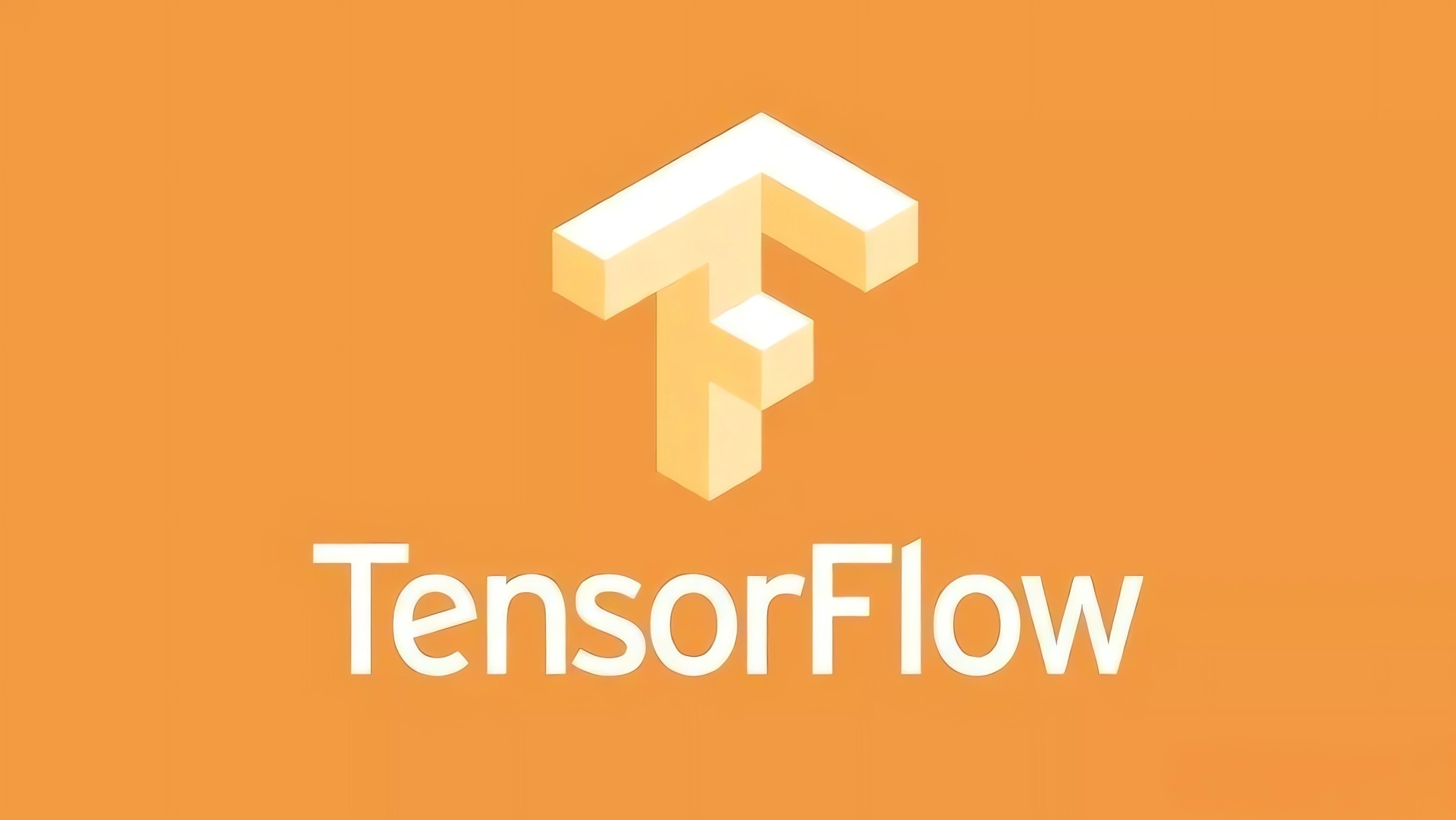TensorFlow 中的内核操作完全用 C ++编写， 以提高效率。 但是用 C++编写 TensorFlow 内核的话可能会非常痛苦。 因此， 在花费数小时实现属于自己的内核之前， 你也许需要先实现一个操作的原型， 尽管这样的效率会很低。 通过 `tf.py_func()` 你可以将任何一个 python 源代码转换为 TensorFlow 的操作。

``````import numpy as np
import tensorflow as tf
import uuid

def relu(inputs):
# Define the op in python
def _relu(x):
return np.maximum(x, 0.)

# Define the op's gradient in python
return np.float32(x > 0)

x = op.inputs

# Register the gradient with a unique id

# Override the gradient of the custom op
g = tf.get_default_graph()
output = tf.py_func(_relu, [inputs], tf.float32)
return output
``````

``````x = tf.random_normal()
y = relu(x * x)

with tf.Session():
diff = tf.test.compute_gradient_error(x, , y, )
print(diff)
``````

`compute_gradient_error()` 数值化地计算梯度， 返回与理论上的梯度的差别， 我们所期望的是一个非常小的差别。 注意到我们的这种实现是非常低效率的， 这仅仅在实现模型原型的时候起作用， 因为 python 代码并不能并行化而且不能在 GPU 上运算（导致速度很慢）。 一旦你确定了你的 idea， 你就需要用 C++重写其内核。 在实践中， 我们一般在 Tensorboard 中用 python 操作进行可视化。 如果你是在构建一个图片分类模型， 而且想要在训练过程中可视化你的模型预测， 那么 TF 允许你通过 `tf.summary.image()` 函数进行图片的可视化。

``````image = tf.placeholder(tf.float32)
tf.summary.image("image", image)
``````

``````import io
import matplotlib.pyplot as plt
import numpy as np
import PIL
import tensorflow as tf

def visualize_labeled_images(images, labels, max_outputs=3, name="image"):
def _visualize_image(image, label):
# Do the actual drawing in python
fig = plt.figure(figsize=(3, 3), dpi=80)
ax.imshow(image[::-1,...])
ax.text(0, 0, str(label),
horizontalalignment="left",
verticalalignment="top")
fig.canvas.draw()

# Write the plot as a memory file.
buf = io.BytesIO()
data = fig.savefig(buf, format="png")
buf.seek(0)

# Read the image and convert to numpy array
img = PIL.Image.open(buf)
return np.array(img.getdata()).reshape(img.size, img.size, -1)

def _visualize_images(images, labels):
# Only display the given number of examples in the batch
outputs = []
for i in range(max_outputs):
output = _visualize_image(images[i], labels[i])
outputs.append(output)
return np.array(outputs, dtype=np.uint8)

# Run the python op.
figs = tf.py_func(_visualize_images, [images, labels], tf.uint8)
return tf.summary.image(name, figs)
``````